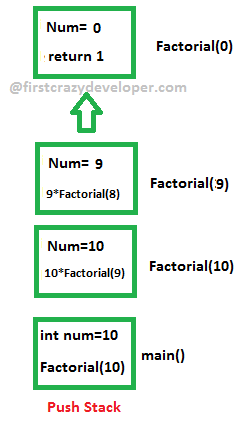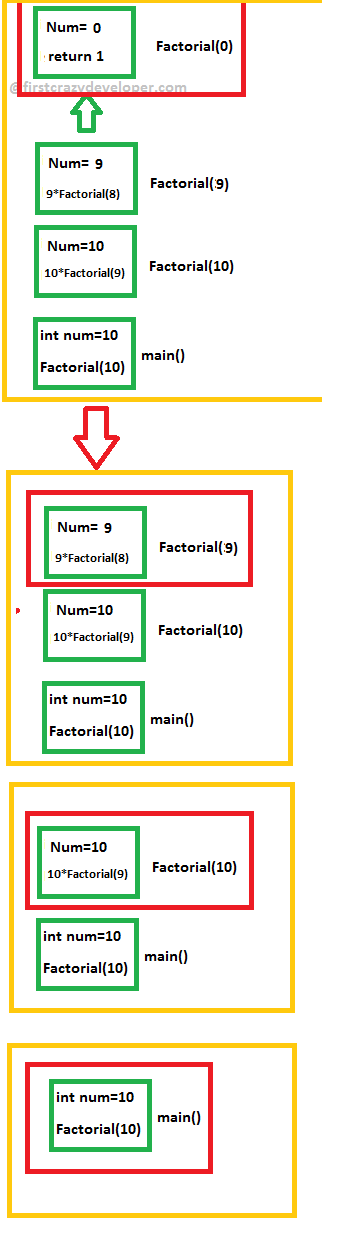© 2021 - First Crazy Developer (Abhishek Kumar)

###Recursive Function & Data Structure

Hi friends, today we will discuss one of the famous function and their data structure. We have an important function in our programming language called
"Recursive Functions". There are lots of question related this function so first we will answer these question then start further discussion about this function and their importance in our programming language.

First we have an important question "What is meant by recursive function?"

Ans: A recursive function (DEF) is a function which either calls itself or is in a potential cycle of function calls. As the definition specifies, there are two types of recursive functions. Consider a function which calls itself: we call this type of recursion immediate recursion.
(Reference : Google)

Next question we have "What is the base case?"

Ans: The base case, or halting case, of a function is the problem that we know the answer to that can be solved without any more recursive calls. The base case is what stops the recursion from continuing on forever. Every recursive function must have at least one base case (many functions have more than one).
(Reference : Google)

Next question we have "What do you mean by recursive algorithm?"

Ans: A recursive algorithm is an algorithm which calls itself with "smaller (or simpler)" input values, and which obtains the result for the current input by applying simple operations to the returned value for the smaller (or simpler) input.
(Reference : Google)

Last but not least one of the favourite question "What is a recursive search?"

Ans: Recursion in computer science is a method where the solution to a problem depends on solutions to smaller instances of the same problem (as opposed to iteration). The approach can be applied to many types of problems, and recursion is one of the central ideas of computer science.
(Reference : Google)

Now we went through most of the popular question regarding recursive in programming. We move ahead and start our discussion related this topic. In simple language we can say "A recursive function is one which calls itself or Recursion is an expression directly or indirectly referencing itself.” First we start with "when we should use recursive function in our program?"

Most common use of recursive function are:

1. Sorting
2. Searching
3. Map
4. Fold
5. Filter

If you ask to me about recursive function then I want to say that "Recursion is a method of solving problems based on the divide and conquer mentality. The basic idea is that you take the original problem and divide it into smaller (more easily solved) instances of itself, solve those smaller instances (usually by using the same algorithm again) and then reassemble them into the final solution."

Here after lots of discussion we have a question "How can we write for recursive method?"

Before going to start sample code I will like to discuss one of my experience of interview. Some years ago an interviewer asked me a question - "How can we print 1 to 10 number without using any loop?"

Do you have any idea? In this question we have a sample code to use recursive function in our program.

Look at the following sample code:

1. static void Printnumber(int MinValue,int MaxValue)
2. {
3. if(MaxValue>= MinValue)
4. {
5. Console.Write("{0} ", MinValue);
6. Printnumber(MinValue + 1, MaxValue);
7. }
8. }

In the above code we can see there is a method named "Printnumber" with two parameters. If MaxValue is greater than equal to MinValue then we print the MinValue and then again call the function itself with two parameters till given condition is true. As per my understanding this is the simplest example of recursive function. With this example you will (hopefully) understand it.

After the basic example we move ahead and look some more cases where we can use this function like:

1. Factorial
2. Fibonacci
3. Euclid's GCD (greatest common denominator)
4. Fourier Transform

We will not go through all the above cases but I will like to touch "Fibonacci" because it is again a most popular question for candidate. Here we will use recursive function to print the Fibonacci series in our program.

Look at the following sample code:

1. static void FibonacciSeries(int a, int b, int counter, int len)
2. {
3. if (counter <= len)
4. {
5. Console.Write("{0} ", a);
6. FibonacciSeries(b, a + b, counter + 1, len);
7. }
8. }

In the above code we can see how we can use recursive function to print Fibonacci series.

Now after lots of discussion about recursive function we move ahead and will look into "Recursive data structures". If you worked with collection then definitely will be used Binary Tree. If we will examine that structure then we will see that a Binary Tree can be defined recursively. If we have to traverse or search a node into tree then due to structure of binary tree recursive function help us to easily play with binary tree. I went through a very good example of "Graphical BinaryTree" with use of recursive function.

Please view this link (http://www.codeproject.com/Articles/334773/Graphical-BinaryTrees) for more details about this example.

To call a recursive function, push arguments and a return value on the stack, and call it like any other function. It returns back the same way as well.

Stack Overflow

While stacks are generally large, they don't occupy all of memory. It is possible to run out of stack space. For example, consider the code we had for factorial.

1. int fact( int n ) {
2. if ( n == 0 )
3. return 1 ;
4. else
5. return fact( n - 1 ) * n ;
6. }

Suppose fact(-1) is called. Then, the base case is never reached (well, it might be reached once we decrement so far that n wraps around to 0 again). This causes one stack frame after another to be pushed. Once the stack limit has been reached, we enter into invalid memory addresses, and the operating system takes over and kills your programming, telling you your program has a stack overflow.

Probably the most common cause of stack overflow is a recursive function that doesn't hit the base case soon enough. For fans of recursion, this can be a problem, so just keep that in mind.

Some languages (say, ML) can convert certain kinds of recursive functions (called "tail-recursive" functions) into loops, so that only a constant amount of space is used.

Now move ahead and again with some example we will understand the stack memory when we use recursive function in our program.

Call Stacks, Recursion, and Stack Frames

A call stack is a data structure used by the program to store information about the active subroutines (like functions in C++ or methods in Java) in a program. The main reason for having a call stack is so that the program can keep track of where a subroutine should return control to once it finishes executing. For example, suppose we have a method “CreateBox” which calls another method “CreateLine” in 4 different places. If the program has finished executing the method CreateLine, then it needs to know where in the CreateBox method it needs to return to. This is why the program uses a call stack – so that it can keep track of these details.

A call stack is composed of stack frames

A stack frame is a part of the call stack, and a new stack frame is created every time a subroutine is called. So, in our recursive Factorial method above, a new stack frame is created every time the method is called. The stack frame is used to store all of the variables for one invocation of a routine. So, remember that a call stack is basically a stack of stack frames.

Stack Frames in Recursion

A diagram of how the stack frames work in recursion will really help to clarify things – so let’s take a look at one. Let’s suppose that we try to find the Factorial of "10" using the function that we created above (so "Num" is equal to 10), this is what the stack frames would look like:

Look at the following code:

1. public static int Factorial(int Num)
2. {
3. if (Num == 0)
4. return 1;
5. else
6. {
7. return Num * Factorial(Num - 1);
8. }
9. }
10. static void Main(string[] args)
11. {
12. int num = 10;
13. Console.WriteLine(Factorial(num).ToString());
14. Console.ReadKey();
15. }

In the above code we used recursive function for Factorial. Now just think what happen in memory when we will execute the above code. First push the stack till provided condition in recursive function method defined.

Look at the following image:In the above image we can see when we execute the above method, in memory first we push 10 stacks then after in sequence recursive function pop the stack and return the result into main program.

Look at the following image:Happy reading!!!

Abhishek Kumar

Happy reading!!!

Abhishek KumarHome Page 11 December 2015